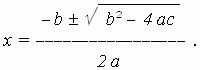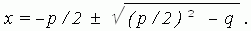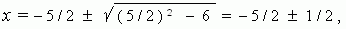# Solution of a quadratic equation

Formulas for solution of non-reduced

In general case of a non-reduced quadratic equation:

ax 2 + bx + c = 0 ,

its roots are found by the formula:If to divide all terms of a non-reduced quadratic equation by a (is it possible ?),  and to sign b / a = p and c / a = q , then we’ll receive the reduced quadratic equation:

x 2 + px + q = 0 ,

roots of which are calculated by the formula:E x a m p l e . x 2 + 5 x + 6 = 0 . Here p = 5, q = 6. Then we have:hence, x 1 = – 5 / 2 + 1 / 2 = – 2 , x 2 = – 5 / 2 – 1 / 2 = 3